# NMF and its Applications ¶

### Non-negative Matrix Factorization¶

##### NMF¶

Given a data matrix $A\in \mathbb R_+^{n\times m}$ and a natural number $k$, the NMF problem statement is to find non-negative matrices $W\in \mathbb R_+^{n\times k}$ and $H\in \mathbb R_+^{k\times m}$ that minimize the distance $$F (W,H) = \|A-WH^T\|^2_F$$

In other words, we want to express each column of $A$ as positive linear combinations of the columns of $W$, since $$A\sim WH^T\implies A_{:,i} \sim H_{i,1}W_{:,1} + H_{i,2}W_{:,2} + \dots + H_{i,k}W_{:,k}$$

Usually, $k$ is way smaller than $n$, so this is a way to compress the data in $A$ into few components.

##### Algorithms¶

There are a lot of algorithms to compute a NMF, but they can be divided into three categories:

• MU, Multiplicative Update
• ALS, Alternating Least Squares
##### MU¶

The Multiplicative Update method is an iterative Gradient Descend method that uses the normal equations associated with the problem $$W^TA\sim (W^TW)H^T \qquad AH \sim W(H^TH)$$

The update operations are

$$H = H \,\,.*\,\, \left[ W^TA\,\, ./\,\, (W^TWH^T + \epsilon I)\right]$$ $$W = W \,\,.*\,\, \left[ AH\,\, ./\,\, (WH^TH + \epsilon I)\right]$$

where $\epsilon$ assures us the positivity of the matrix at the denominator.

Each update makes the error function decrease, but it doesn't always converge to a stationary point. The MU method tends to produce sparse solutions, since each update retains the zero elements.

##### PG¶

We can use a Projected Gradient method, where the updates of $H,W$ are contemporaneous through the rule

$$(W,H) = (W,H) - \alpha(\nabla_W F(W,H), \nabla_H F(W,H))$$$$W = W_+ \qquad H = H_+$$

in which $\alpha$ changes at every step.

After the first iteration, $(W,H)$ will usually converge to the stationary point $(0,0)$, but it's sufficient to find an initial couple $(W_0,H_0)$ satisfying $$\|A\|_F > \|A-W_0H_0^T\|_F$$

It's not guaranteed its convergence to a stationary point.

##### ALS¶

The Alternating Least Square method solves iteratively the convex problems associated to NMF without positivity constraints. $$H =\arg\min_{X\in \mathbb R^{m\times k}} \|A-WX^T\|^2_F \qquad \text{make }H\text{ nonnegative}$$ $$W =\arg\min_{X\in \mathbb R^{n\times k}} \|A-XH^T\|^2_F \qquad \text{make }W\text{ nonnegative}$$

An other option is to retain the positivity constraint in the convex problems. It assures that every limit point of the sequence generated by the algorithm is a local minimum, but the computational cost rises substantially.

It's the algorithm used by the Python sklearn NMF and the Matlab nnmf.

In the ALS setting, two methods are usually used to solve the internal convex problem:

##### Internal PG¶

$$H =\arg\min_{X\in \mathbb R^{m\times k}} \|A-WX^T\|^2_F \qquad H = H_+$$ $$W =\arg\min_{X\in \mathbb R^{n\times k}} \|A-XH^T\|^2_F \qquad W = W_+$$

##### Internal CD¶

A Coordinate Descent method, in which the entries of the matrices are computed one by one.

Let's see a comparison between the two methods.

First, we compute the errors of the two metods when the matrix dimension rises.

In :
from sklearn.decomposition import NMF
import numpy as np
import warnings
warnings.filterwarnings('ignore')
model = NMF(n_components=2, init='random', solver='pg', random_state=np.random.randint(100), tol = 1e-5)
model2 = NMF(n_components=2, init='random', solver='cd', random_state=np.random.randint(100), tol = 1e-5)
e1 = np.zeros(50)
e2 = np.zeros(50)
for n in range(5,55):
A = np.random.rand(n,n)
model.fit(A)
model2.fit(A)
e1[n-5] = model.reconstruction_err_
e2[n-5] = model2.reconstruction_err_
print('done')

done


Then we plot the difference in a graph.

In :
%matplotlib inline
import matplotlib.pyplot as pl
pl.plot(e2-e1);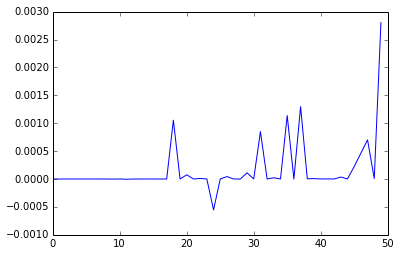On low dimensions, there's little difference, but the PG methods are usually deprecated since the error may not decrease at each step.

### Applications ¶

The NMF is used in several areas as a clustering or compression method. For example it appears in

• Image Processing
• Sound Processing
• Text Mining
##### Clustering¶

Given $m$ points $x_1,x_2,\dots,x_m$ in $\mathbb R^n$, we may want to cluster them.

A translation doesn't change the overall structure of the points, so we can suppose to work on the positive orthant $\mathbb R_+^n$.

If we set $x_i$ as the columns of $A$ and perform an NMF on it, then $$A\sim WH^T$$ where each $x_i$ is a positive linear combination of the columns of $W$.

If we see the columns of $W$ as points in $\mathbb R_+^n$, then the factorization tend to identify them as the centroids of the clusters, so a point $x_i$ belong in the cluster of the column $W_{:,j}$ iff the coefficient $H_{ij}$ in $$x_{i} \sim H_{i,1}W_{:,1} + H_{i,2}W_{:,2} + \dots + H_{i,k}W_{:,k}$$ is the maximum of the row $H_{i,:}$

Let's generate 90 points on the plain, within three distinct clusters.

In :
from mpl_toolkits.mplot3d import Axes3D
A = np.concatenate((np.array( [ [1,3,10] + np.random.rand(3)-.5 for i in range(30) ] ),
np.array( [ [3,7,2] + np.random.rand(3)-.5 for i in range(30) ] ),
np.array( [ [5,1,1] + np.random.rand(3)-.5 for i in range(30) ] )),axis = 0)
fig = pl.figure()
ax = Axes3D(fig)
ax.scatter(A[:,0],A[:,1],A[:,2])
pl.show()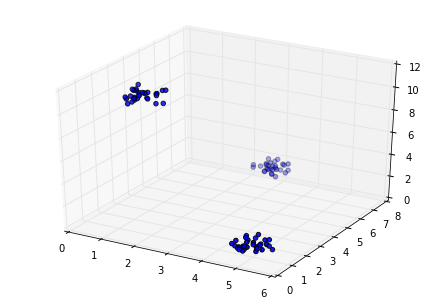Let's now associate each point with the relative centroid using NMF.

In :
model = NMF(n_components=3, init='random', random_state=np.random.randint(100), tol = 1e-10)
model.fit(A.T)
H = model.components_
h = np.argmax(H,0)
fig = pl.figure()
ax = Axes3D(fig)
ax.scatter(A[h==0][:,0],A[h==0][:,1],A[h==0][:,2],c='r');
ax.scatter(A[h==1][:,0],A[h==1][:,1],A[h==1][:,2],c='b');
ax.scatter(A[h==2][:,0],A[h==2][:,1],A[h==2][:,2],c='y');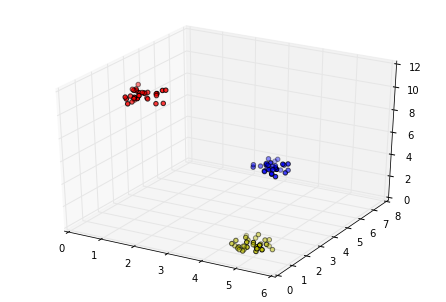Some problem arises when the centroids of the clusters are linearly dependent.

In :
A = np.concatenate((np.array( [ [1,1,1] + np.random.rand(3)-.5 for i in range(30) ] ),
np.array( [ [5,5,5] + np.random.rand(3)-.5 for i in range(30) ] ),
np.array( [ [11,11,11] + 2*np.random.rand(3)-1 for i in range(30) ] )),axis = 0)
model.fit(A.T)
H = model.components_
h = np.argmax(H,0)
fig = pl.figure()
ax = Axes3D(fig)
ax.scatter(A[h==0][:,0],A[h==0][:,1],A[h==0][:,2],c='r');
ax.scatter(A[h==1][:,0],A[h==1][:,1],A[h==1][:,2],c='b');
ax.scatter(A[h==2][:,0],A[h==2][:,1],A[h==2][:,2],c='y');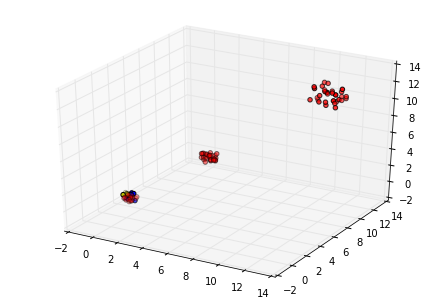It can be solved by computing a similarity matrix of the points, and applying the NMF on it.

In :
M = [[np.exp( - np.linalg.norm(v-w) ) for v in A] for w in A]
model.fit(M)
H = model.components_
h = np.argmax(H,0)
fig = pl.figure()
ax = Axes3D(fig)
ax.scatter(A[h==0][:,0],A[h==0][:,1],A[h==0][:,2],c='r');
ax.scatter(A[h==1][:,0],A[h==1][:,1],A[h==1][:,2],c='b');
ax.scatter(A[h==2][:,0],A[h==2][:,1],A[h==2][:,2],c='y');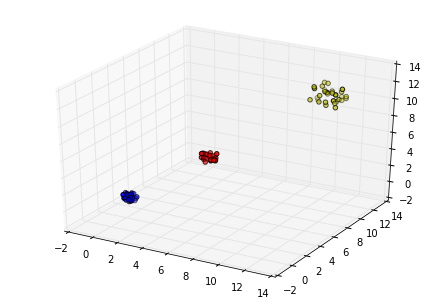This last method increases the complexity, but solves a lot of instability problems of a classical NMF.

For example now the problem is invariant under translations and rotations, and it works since, if two points are near, then also their distances from the other points are similar.

It also can be modified into a symmetric NMF problem: given $S$ a symmetric nonnegative matrix, find a non-negative matrix $W\in \mathbb R_+^{n\times k}$ that minimizes the distance $$G (W) = \|S-WW^T\|^2_F$$ This problem is proved to be strictly related to a spectral clustering.

In :
import math
A = np.concatenate((np.array([[2*math.cos(math.pi*(math.cos(i)/2)),2*math.sin(math.pi*(math.cos(i)/2))]
for i in range(90)]),
np.array([[2*math.cos(math.pi*(1+math.cos(i)/2)),2+2*math.sin(math.pi*(1+math.cos(i)/2))]
for i in range(90)])),  axis=0 )
model = NMF(n_components=2, init='random', random_state=np.random.randint(100), tol = 1e-5)
M = [[np.exp( - np.linalg.norm(v-w) ) for v in A] for w in A]
model.fit(M)
H = model.components_
h = np.argmax(H,0)
pl.scatter(A[h==0][:,0],A[h==0][:,1],c='r');
pl.scatter(A[h==1][:,0],A[h==1][:,1],c='b');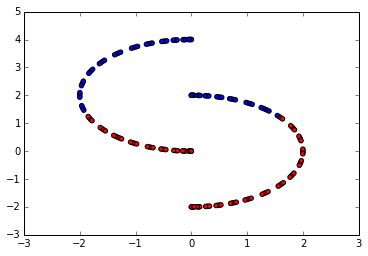##### Principal Image Components¶

Given a set of images, we can decompose them in components through the NMF.

If we vectorize the images and put them as columns of a matrix $A$, and perform an NMF $$A \sim WH^T$$ Then we'll likely find the components in common between the images in the columns of $W$ , so that their combinations reconstruct the original images.

In this case, the sparsity is required for $H$ and $W$, since the columns of $W$ will be small fragments of the images, and each original image will be composed by few components of $W$.

For example, given a set of faces, NMF recognizes eyes, noses, eyebrows, lips, etc. as shown by the experiment performed on the original paper by Lee & Seung(1999).We'll return later on this theme.

##### Text Mining¶

A last application of the NMF regards the text mining.

Given a set of documents, we can count the words with repetitions, and associate to each text the relative frequency of the words as an array. We can now perform the NMF on the matrix that has these arrays as columns, obtaining $$A\sim WH^T$$

If two words are related to the same subject, then they'll often appear in the same documents.

The NMF manages to identify large groups of words that appears togheter, so it can distinguish beetween different subjects, without having them beforehand.

An other experiment, conducted by Lee & Seung on an english encyclopedia, shows that the NMF can also discover when the same word belongs to different subjects (in this case, the word 'lead')### NMF and Permutations¶

Even if NMF is used to decompose images, it can't recognise a common feature in different images if it is located in different places. In other words, NMF is not invariant under space transformations, so the input data must always be pre-calibrated and adjusted.

In order to solve such a problem, we'll modify the contents of NMF matrices, but without touching their structure, so that the final form will again be $$\min_{W,H} \|A-W*H^T\|_F$$ where $W$ will contain as before the wanted components, whereas $H$ will contain informations on where to find the component of $W$ inside every original image in $A$.

##### The Permutation Class¶

The elements of $H$ will belong to the permutation class.

Each istance of this class is composed by two numbers $$\tau \equiv [r,s] \qquad r\in \mathbb R\quad s\in \frac{\mathbb Z}{n\mathbb Z}$$

The permutation is called "positive" if $r\ge 0$.

The main feature of $\tau$ is that we can apply it to a vector $v\in \mathbb R^n$: $$\tau(v) = [r,s](v) = r\sigma_s(v)$$

where the $\sigma_s$ is the cyclic shift operator on $\mathbb R^n$, that is $$\sigma_s(v) = w\in \mathbb R^n \implies w_i = v_{j}\,:\, j\equiv i+s\pmod n$$

In :
import permutation

##### The Permutation Matrix Class¶

We introduce a wrapper for matrices of permutations, the permutation_matrix class. We also need to define an operation between real and permutation matrices, called the Diamond Operator.

Given a real matrix $W\in\mathbb R^{n\times k}$ and a permutation matrix $H$ of dimension ${k\times m}$, it is defined as $$(W\diamond H)_{:,i} := \sum_{j=1}^k H_{ji}(W_{:,j})$$ In other words, the $i$-th column of the diamond product is a linear combination of permutations of $W$ columns, determined by the elements of the $H$ $i$-th column.

In :
import permutation_matrix as pm

##### PermNMF¶

We can now state the PermNMF problem.

Given a matrix $A$ in $\mathbb R_+^{n \times m}$, we want to find a permutation matrix $H$ of dimension ${m\times k}$ with nonnegatice coefficients, and a matrix $W$ in $\mathbb R_+^{n\times k}$ that minimize $$F(W,H) = \| A - W \diamond H^T\|_F^2$$

In order to solve this problem, we'll move step by step.

###### Single PermNNLS¶

Given two vectors $v,w\in \mathbb R^n$, find the positive permutation $\tau\equiv[r,s]$ that minimizes $$\| v - \tau(w)\|$$

If we fix $s$, then we can solve for $r$, because $$r_s := \arg\min_{r\in \mathbb R_+}\|v - r\sigma_s(w)\|^2 = \max\left\{0,\frac{v^T\sigma_s(w)}{\|w\|^2}\right\}$$ $$\|v - r_s\sigma_s(w)\|^2 = \|v\|^2 - \frac{(v^T\sigma_s(w))^2}{\|w\|^2}$$ The problem is thus equivalent to find the greatest component of $v^TC$, with $C$ the real nonnegative and circulant matrix with $w$ as first row. It can be computed with some FFT.

The Single PermNNLS Algorithm is

In :
import auxil
import numpy as np

def Simple_PermNNLS( v,w ):
"""
solves min_s ||v-s(w)|| with fft
"""

z = auxil.circulant_product(w,v)
n = len(v)
r = np.argmax(z)
M = z[r]
if M < 0:
c = 0
else:
c = M / np.linalg.norm(w)**2
return permutation.permutation(c,-r,n)


Let's now complicate the problem.

##### Multiple PermNNLS¶

Given a vector $v\in \mathbb R^n$, and a set of vectors $w_1,w_2,\dots,w_k\in \mathbb R^n$, find the positive permutations $\tau_1,\dots,\tau_k$ that minimize the quantity $$\|v - (\tau_1(w_1) + \tau_2(w_2) + \dots + \tau_k(w_k))\|$$

We're looking for the best linear positive combination of the vectors $w_i$ shifted that returns the original vector $v$. If we call $W$ the matrix with $w_i$ as columns, and $x$ the vector of $\tau_i$, then we can rewrite the problem in a compact way as $$\min_{x} \|v-W\diamond x\|^2 \qquad v\in\mathbb R^n \quad W \in \mathbb R^{n\times k}$$

A way to solve this problem is using the precedent algorithm in an alternated fashion: if we fix $\tau_2,\tau_3,\dots,\tau_k$, then it reduces to a Single PermNNLS problem on $\tau_1$, and we know how to solve it exactly.

The algorithm becomes

In :
import copy
def Multiple_PermNNLS( v,W,P ):
"""
returns the array of permutations obtained through Simple_PermNNLS on the columns of W
on the problem min||v-W*P|| with an alternating algorithm.
Its inputs are an array, a matrix, and an initial array of permutations
"""
(s,m) = pm.size(W)
PP = np.array(P).reshape(( m, 1))
w = pm.permutation_matrix(PP).rmul(W)
Q = copy.deepcopy(P)
M = copy.deepcopy(P)
mini = np.linalg.norm(v-w)
for j in np.arange(10):
for i in np.arange(m):
w = w - Q[i].apply_per(W[:,[i]])
Q[i] = Simple_PermNNLS(v-w,W[:,[i]])
w = w + Q[i].apply_per(W[:,[i]])

c = np.linalg.norm(v-w)
if c < mini :
M = copy.deepcopy(Q)
mini = c
return M

##### Matrix PermNNLS¶

Given $A\in \mathbb R^{n\times m}$ and $W\in \mathbb R^{n\times k}$, find the permutation matrix $H$ of dimension ${k\times m}$ that minimize $$F(X) = \|A-W\diamond X^T\|^2_F$$

Like the normal NMF, it can be decomposed into smaller problems $$\|A-W\diamond X^T\|^2_F=\sum_{i=1}^m \|A_{:,i}-W\diamond (X^T)_{:,i}\|^2$$ so it can be solved with the Multiple Permutation NNLS algorithm on each column.

The Algorithm is

In :
def Matrix_PermNNLS( V,W,H ):
"""
returns the matrix of permutations obtained through
Multiple_PermNNLS on the problem min_X ||V-W*X'||
"""
(n,m) = pm.size(H)

P = copy.deepcopy(H.mm)
Q = [  Multiple_PermNNLS(V[:,[i]],W,P[i])  for i in np.arange(n)   ]

return pm.permutation_matrix(Q)


Now we can sum it up and return to the original problem

##### ALS - PermNMF¶

We use an Alternating method to solve the problem.

The update rule for $W$ will be a Projected Gradient for simplicity, whereas the update rule of $H$ is performed through the Matrix PermNNLS algorithm.

$$H = \text{Matrix_PermNNLS} ( A,W,H )$$$$W = \text{PG} (A,W,H)$$

We will stop when the error becames too small, or when we obtain the same couple $(W,H)$ at the end of a cycle, meaning we entered in a loop.

In :
import time
def PermALS(A,k,x,y):
"""
Perform an ALS algorithm for the PermNMF problem
"""
(r,s) = pm.size(A)
W = np.random.rand(r,k)
H = pm.randpermutmat(s,k,r)
err = np.zeros(2000)
for i in np.arange(1000):
W = auxil.W_gd(A,W,H)
err[2*i] = np.linalg.norm(A-(~H).rmul(W),'fro')
if err[2*i]<.0001:
break
H = Matrix_PermNNLS(A,W,H)
err[2*i+1] = np.linalg.norm(A-(~H).rmul(W),'fro')
if err[2*i+1]<.0001:
break
if (i>0 and err[2*i+1]==err[2*i-1]):
err[2*i+2:] = err[2*i+1]
break
auxil.show_comp(A,W,H,x,y)
time.sleep(.5)
return [W,H,err]

##### Experiment¶

Let's eventually try with an experiment: we'll generate some simple patterns and randomly translate and sum them.

In [ ]:
%matplotlib inline
A = np.zeros((30,30))
A=1
B = np.zeros((30,30))
B[1:6,1:6]=1
C = auxil.Matrix_Combination(10,[A,A,B,B])
[W,H,err]=PermALS(C,4,30,30);
# If it doesn't work, try again. The initial point is randomic